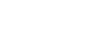# [Glossary] PW mode measurement

The glossary of “Auto Measure”

• HR (bpm)：Heart rate
• PSV (cm/s)：Peak systolic velocity
• EDV (cm/s)：End diastolic velocity
• PI：Pulsatility index (of Gosling)
• PI = (PSV–EDV)/MV
• MV (cm/s)：Mean velocity
• RI：Resistance index (of Pourcelot)
• RI = (PSV–EDV)/PSV
• VTI (cm)：Velocity-time integral
• TAV Max (cm/s)：The maximum of time-averaged velocity
• S/B：The average RI of a cycle
• SD：Systolic/Diastolic Ratio
• SD = PSV/EDV
• ACCL (cm/s²)：The acceleration index
• ACCL = (PSV – EDV)/ACCT
• ACCT (s)：The time from the lowest (EDV) to the highest (PSV)
• VFM (ml/min)：Volume flow per minute
• VFM Max (ml/min)：The maximum of volume flow per minute
• Diam (mm) ：Diameter

⚠VFM, VFM Max, Diam values will be shown when you are in the frozen state.

The glossary of measure tool “PW V, T, HR”

• T (s)：Time
• HR (bpm)：Heart rate
• Range (cm/s)：The range of flow velocity

The glossary of measure tool “RI, S/D”

• PSV (cm/s)：Peak systolic velocity
• EDV (cm/s)：End diastolic velocity
• S/D：Systolic/Diastolic Ratio
• SD = PSV/EDV
• RI：Resistance index (of Poucelot)
• RI = (PSV–EDV)/PSV

The glossary of measure tool “PI”

• PSV (cm/s)：Peak systolic velocity
• D (cm/s)：End diastolic velocity
• Area (cm²)：Blood vessel cross-sectional area
• Diam (mm)：Diameter
• PI：Pulsatility index (of Gosling)
• PI = (PSV–EDV)/MV
• MV (cm/s)：Mean velocity
• VFM Max (ml/min)：The maximum of volume flow per minute
• TAV Max (cm/s)：The maximum of time-averaged velocity

The glossary of measure tool “VTI”

• VTI (cm)：Velocity-time integral